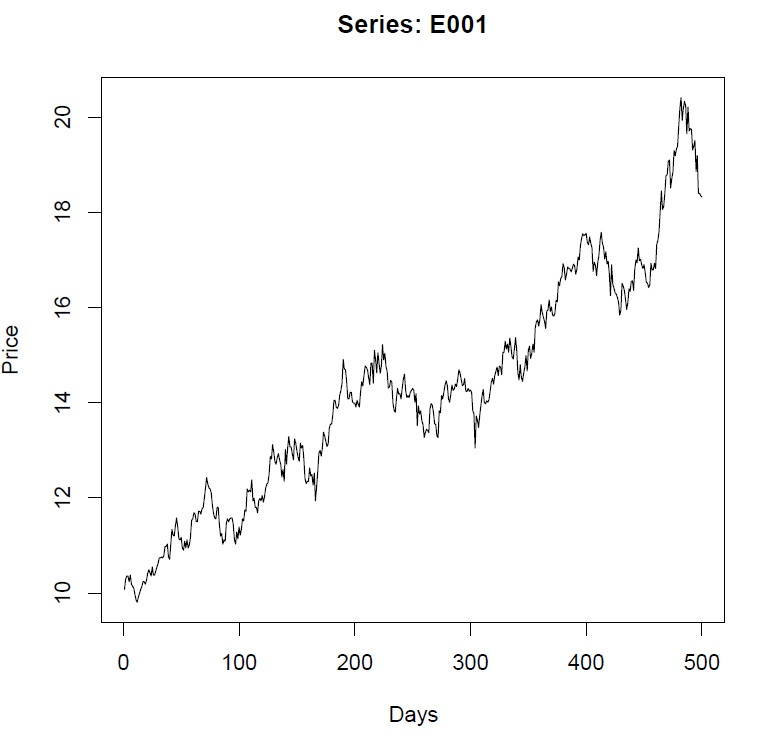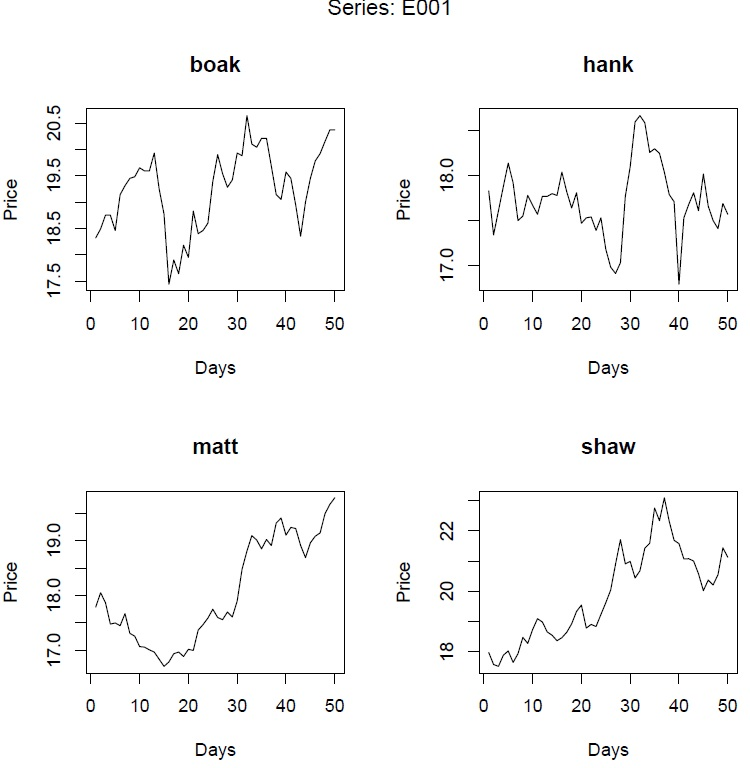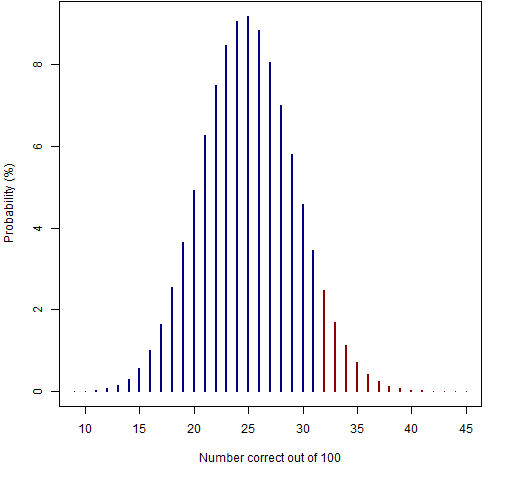02 Jan

# The Technical Analysis Challenge

## The game

You are shown 500 daily prices of an asset (an equity).  The first series is:To go with this, there is a choice of four series of 50 prices each.  One of the choices is the actual subsequent 50 prices; the other three choices are randomly generated.  Here are the choices for the first series:The task is to name the extension that is the actual data.  There are 100 series in all.

## The pictures

There are two ways you can look at the series.  The first way is to have the in-sample and the extensions separate, as above:

The other way is to have the full 550 days together:

If you answer all 100 questions, then the picture shows the probabilities assuming pure guessing.You need to answer at least 32 correct in order to have a p-value less than 5% for the test of being better than guessing.

You may not want to answer all the questions because some of the choices look very  similar.  The R command to get the p-value is:

`1 - pbinom(number.correct, number.answered, p=.25)`

For example if you answer 50 questions, then you need at least 18 correct to have the p-value less than 5%:

```> 1 - pbinom(17, 50, p=.25)
 0.05512336```

## History

This test was done without the answers being available.  The challenge ran from 2003 September 6 to 2003 October 4.  The working paper “The Technical Analysis Challenge” reports the results.

## Data

You can get the actual data from: